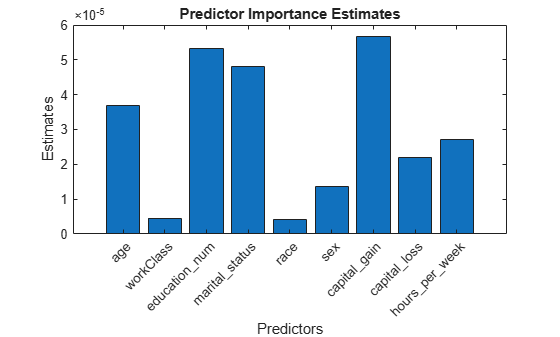Documentation

predictorImportance

Estimates of predictor importance for classification tree

Syntax

imp = predictorImportance(tree)

Description

imp = predictorImportance(tree) computes estimates of predictor importance for tree by summing changes in the risk due to splits on every predictor and dividing the sum by the number of branch nodes.

Input Arguments

 tree A classification tree created by fitctree, or by the compact method.

Output Arguments

 imp A row vector with the same number of elements as the number of predictors (columns) in tree.X. The entries are the estimates of predictor importance, with 0 representing the smallest possible importance.

Examples

expand all

Load Fisher's iris data set.

Grow a classification tree.

Mdl = fitctree(meas,species);

Compute predictor importance estimates for all predictor variables.

imp = predictorImportance(Mdl)
imp = 1×4

0         0    0.0907    0.0682

The first two elements of imp are zero. Therefore, the first two predictors do not enter into Mdl calculations for classifying irises.

Estimates of predictor importance do not depend on the order of predictors if you use surrogate splits, but do depend on the order if you do not use surrogate splits.

Permute the order of the data columns in the previous example, grow another classification tree, and then compute predictor importance estimates.

measPerm  = meas(:,[4 1 3 2]);
MdlPerm = fitctree(measPerm,species);
impPerm = predictorImportance(MdlPerm)
impPerm = 1×4

0.1515         0    0.0074         0

The estimates of predictor importance are not a permutation of imp.

Load Fisher's iris data set.

Grow a classification tree. Specify usage of surrogate splits.

Mdl = fitctree(meas,species,'Surrogate','on');

Compute predictor importance estimates for all predictor variables.

imp = predictorImportance(Mdl)
imp = 1×4

0.0791    0.0374    0.1530    0.1529

All predictors have some importance. The first two predictors are less important than the final two.

Permute the order of the data columns in the previous example, grow another classification tree specifying usage of surrogate splits, and then compute predictor importance estimates.

measPerm  = meas(:,[4 1 3 2]);
MdlPerm = fitctree(measPerm,species,'Surrogate','on');
impPerm = predictorImportance(MdlPerm)
impPerm = 1×4

0.1529    0.0791    0.1530    0.0374

The estimates of predictor importance are a permutation of imp.

Load the census1994 data set. Consider a model that predicts a person's salary category given their age, working class, education level, martial status, race, sex, capital gain and loss, and number of working hours per week.

'sex','capital_gain','capital_loss','hours_per_week','salary'});

Display the number of categories represented in the categorical variables using summary.

summary(X)
Variables:

age: 32561x1 double

Values:

Min        17
Median     37
Max        90

workClass: 32561x1 categorical

Values:

Federal-gov              960
Local-gov               2093
Never-worked               7
Private                22696
Self-emp-inc            1116
Self-emp-not-inc        2541
State-gov               1298
Without-pay               14
NumMissing              1836

education_num: 32561x1 double

Values:

Min              1
Median          10
Max             16

marital_status: 32561x1 categorical

Values:

Divorced                       4443
Married-AF-spouse                23
Married-civ-spouse            14976
Married-spouse-absent           418
Never-married                 10683
Separated                      1025
Widowed                         993

race: 32561x1 categorical

Values:

Amer-Indian-Eskimo      311
Asian-Pac-Islander     1039
Black                  3124
Other                   271
White                 27816

sex: 32561x1 categorical

Values:

Female    10771
Male      21790

capital_gain: 32561x1 double

Values:

Min               0
Median            0
Max           99999

capital_loss: 32561x1 double

Values:

Min               0
Median            0
Max            4356

hours_per_week: 32561x1 double

Values:

Min               1
Median           40
Max              99

salary: 32561x1 categorical

Values:

<=50K     24720
>50K       7841

Because there are few categories represented in the categorical variables compared to levels in the continuous variables, the standard CART, predictor-splitting algorithm prefers splitting a continuous predictor over the categorical variables.

Train a classification tree using the entire data set. To grow unbiased trees, specify usage of the curvature test for splitting predictors. Because there are missing observations in the data, specify usage of surrogate splits.

Mdl = fitctree(X,'salary','PredictorSelection','curvature',...
'Surrogate','on');

Estimate predictor importance values by summing changes in the risk due to splits on every predictor and dividing the sum by the number of branch nodes. Compare the estimates using a bar graph.

imp = predictorImportance(Mdl);

figure;
bar(imp);
title('Predictor Importance Estimates');
ylabel('Estimates');
xlabel('Predictors');
h = gca;
h.XTickLabel = Mdl.PredictorNames;
h.XTickLabelRotation = 45;
h.TickLabelInterpreter = 'none';In this case, capital_gain is the most important predictor, followed by education_num.